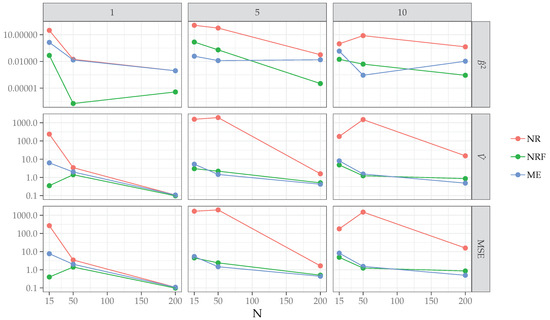Next Article in Journal
Analytical Solutions of Fractional-Order Heat and Wave Equations by the Natural Transform Decomposition Method
Next Article in Special Issue
Model Selection for Non-Negative Tensor Factorization with Minimum Description Length
Previous Article in Journal
Confidential Cooperative Communication with the Trust Degree of Jammer
Previous Article in Special Issue
SIMIT: Subjectively Interesting Motifs in Time Series
Open AccessArticle

# A Maximum Entropy Procedure to Solve Likelihood Equations

byAntonio Calcagnì *,Livio Finos,Gianmarco AltoéandMassimiliano PastoreDepartment of Developmental and Social Psychology, University of Padova, 35131 Padova, Italy
*
Author to whom correspondence should be addressed.
Entropy 2019, 21(6), 596; https://doi.org/10.3390/e21060596
Received: 20 May 2019 / Revised: 13 June 2019 / Accepted: 14 June 2019 / Published: 15 June 2019
(This article belongs to the Special Issue Information-Theoretical Methods in Data Mining)
In this article, we provide initial findings regarding the problem of solving likelihood equations by means of a maximum entropy (ME) approach. Unlike standard procedures that require equating the score function of the maximum likelihood problem at zero, we propose an alternative strategy where the score is instead used as an external informative constraint to the maximization of the convex Shannon’s entropy function. The problem involves the reparameterization of the score parameters as expected values of discrete probability distributions where probabilities need to be estimated. This leads to a simpler situation where parameters are searched in smaller (hyper) simplex space. We assessed our proposal by means of empirical case studies and a simulation study, the latter involving the most critical case of logistic regression under data separation. The results suggested that the maximum entropy reformulation of the score problem solves the likelihood equation problem. Similarly, when maximum likelihood estimation is difficult, as is the case of logistic regression under separation, the maximum entropy proposal achieved results (numerically) comparable to those obtained by the Firth’s bias-corrected approach. Overall, these first findings reveal that a maximum entropy solution can be considered as an alternative technique to solve the likelihood equation. View Full-Text
Show FiguresFigure 1

MDPI and ACS Style

Calcagnì, A.; Finos, L.; Altoé, G.; Pastore, M. A Maximum Entropy Procedure to Solve Likelihood Equations. Entropy 2019, 21, 596.